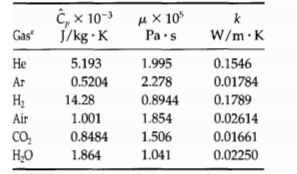### Create an Account

Home / Questions / Computation of the Prandtl numbers for gases at low density a By using the Eucken formula ...

# Computation of the Prandtl numbers for gases at low density a By using the Eucken formula and experimental heat capacity data estimate the Prandtl number at 1 atm and 300K for each of the gases

Computation of the Prandtl numbers for gases at low density. (a) By using the Eucken formula and experimental heat capacity data, estimate the Prandtl number at 1 atm and 300K for each of the gases listed in the table. (b) For the same gases, compute the Prandtl number directly by substituting the following value: of the physical properties into the defining formula Pr = C,p µ/k, and compare the values with the results obtained in (a). All properties are given at low pressure and 300KAug 06 2020 View more View LessSubscribe To Get Solution